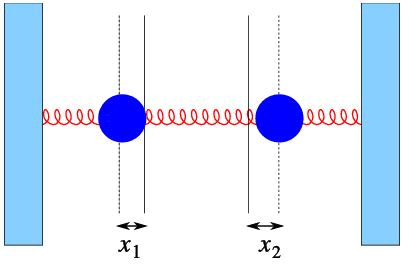Courses

# Eigenvalues and Eigenvectors(includes examples) - Mathematical Methods of Physics, UGC - NET Phy Physics Notes | EduRev

## Physics : Eigenvalues and Eigenvectors(includes examples) - Mathematical Methods of Physics, UGC - NET Phy Physics Notes | EduRev

The document Eigenvalues and Eigenvectors(includes examples) - Mathematical Methods of Physics, UGC - NET Phy Physics Notes | EduRev is a part of the Physics Course Physics for IIT JAM, UGC - NET, CSIR NET.
All you need of Physics at this link: Physics

Eigenvalues and eigenvectors

In understanding the nature of a matrix (or really the linear transformation represented by it) we often would like to understand those vectors that are transformed into themselves, i.e., where

A e = λ e , ( λ  is a constant).           (1)

This equation is called the “eigenvalue problem”; λ is called an eigenvalue and e an eigenvector.

The question is now, obviously, how to determine λ and e. By using a simple trick, we can solve for λ without having to know e. To this end we write

λ x = λ I x = λ 0 0 λ x ,

and we can thus rewrite the eigenvalue problem as

( A − λ I ) x = 0 .                      (2)

We have argued in the previous section that this has only the trivial solution if det( A−λ I) ≠ 0, i.e., x= 0. In order to avoid this we must require

det ( A − λ I ) = 0 .                      (3)

For a two-by-two matrix this is a simple quadratic equation.

Example  5.1:

Find the eigenvalues and eigenvectors of A= 1 2 2 1 .

Solution:

det ( A − λ I ) = 1 − λ 2 2 1 − λ = ( 1 − λ ) 2 − 4 = 0

Solutions are λ= 1± 2=− 1, 3.

Now determine the eigenvalues by solving ( A−λ I) e= 0. For λ 1=− 1 we ﬁnd

2 2 2 2 e 1 e 2 = 0

and thus e 1=− e 2, and e( 1)= c 1 1 . The arbitrary constant can be chosen at will. Some standard choices are 1(simple), 1∕ 2(length 1), etc.

The same algebra for the other eigenvalue leads to e( 2)= d 1− 1 .

If we rewrite

x y = ( x + y ) ∕ 2 1 1 + ( x − y ) ∕ 2 1 − 1 ,

we can easily understand the importantce of the eigenvectors:

A x y = 3 ( x + y ) ∕ 2 1 1 − ( x − y ) ∕ 2 1 − 1 .

Thus the component parallel to ( 1, 1) is stretches by a factor of 3, and the component parallel to (− 1, 1) is inverted (multiplied by − 1)

A physics example

The most important physical example of the role of the eigenvalue problem can be found in the case of coupled oscillators.Figure 1 Two coupled oscillators.

Consider Fig.  1 . There we show the case of two masses, coupled by three springs. We assume that x 1 and x 2 are the distances of the masses from the equilibrium position. At that point we assume the strings are untentioned (neither stretched nor compressed).

The equations of motion take a simple form

m 1 ẍ 1 = − k 1 x 1 + k 2 ( x 2 − x 1 ) = − ( k 1 + k 2 ) x 1 + k 2 x 2 m 2 ẍ 2 = − k 3 x 2 − k 2 ( x 2 − x 1 ) = − ( k 3 + k 2 ) x 2 + k 2 x 1

We now take the masses equal ( m 1= m 2= m), and all the spring constants equal as well ( k 1= k 2= k 3= mω 2. We then ﬁnd that

x ̈ = ω 2 − 2 1 1 − 2 x

This equation can now be solved by writing the standard exponential form, x= e e z t. We then get

z 2 e = ω 2 − 2 1 1 − 2 e

Which is an eigenvalue problem. Write z 2=ω 2λ, and we ﬁnd that λ=− 1,− 3. Thus z=± iω,± i 3ω. The eigenvectors for these two eigenvalues are ( 1, 1)and ( 1,− 1), respectively.

Thus, in all its generality, we ﬁnd using superposition that

x = 1 1 ( A cos ( ω t ) + B sin ( ω t ) ) + 1 − 1 ( C cos ( 3 ω t ) + D sin ( 3 ω t ) )                .(4)

This general motion thus consists of the superposition of motion of the two masses in phase ( x 1= x 2, with frequency ω) and one maximally out of phase ( x 1=− x 1, with frequency 3ω).

Offer running on EduRev: Apply code STAYHOME200 to get INR 200 off on our premium plan EduRev Infinity!

159 docs

,

,

,

,

,

,

,

,

,

,

,

,

,

,

,

,

,

,

,

,

,

,

,

,

;